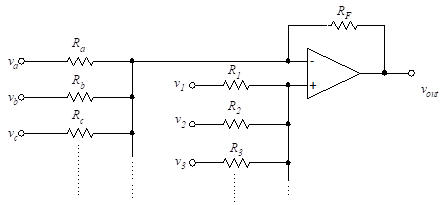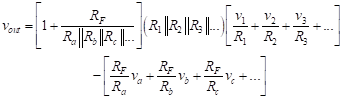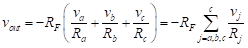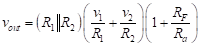# 5. Combined Inverting and non-Inverting Inputs

Combined Inverting and Non-inverting Inputs

The general case of input configuration is a combination of the previous two cases. That is, we allow for both inverting and non-inverting inputs. The general configuration is shown in Figure (13).Figure 13- Inverting and non-inverting inputs

The previous circuits can be considered as special cases of this general problem. The output relationship is found by applying superposition as follows. Equation (29)(29)

is derived by combining Equation (17)(17)

with Equation (25)(25)

Equation (29) represents a general result which will prove useful in analyzing a wide variety of circuits. With equations this complex, it is always a good idea to verify that the units on each side of the equality match. Indeed, the units on both sides of the equality are “volts”.